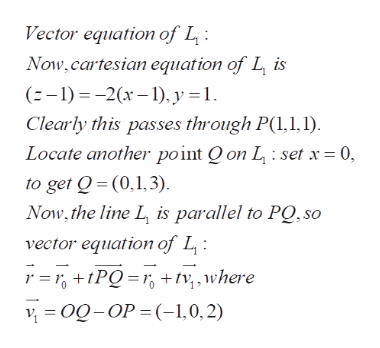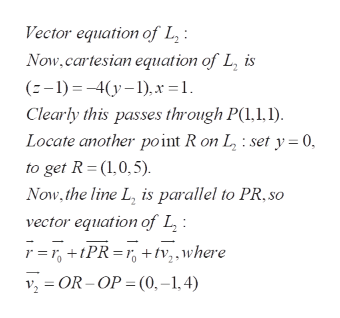# For function z = f(x,y) where f(x,y) = 4−x2-2y2 , we compute that fx(1,1) =−2 and fy(1,1) = −4. We have learned that the partial derivative fx(1, 1) determines a tangent line L1 of graph z = f(x, y) at (1, 1, 1). In particular, the line L1 can be described by a system of two equationsz − 1 = −2(x − 1), y = 1.Similarly, fy(1,1) determines one other tangent line L2 of graph z = f(x,y) at (1, 1, 1), which can be described byz − 1 = −4(y − 1), x = 1.a)  Write the vector equations of L1 and L2, respectively. Specifically, describe the equation of L1 as ⃗r = ⃗r0 + t⃗v1 for some ⃗v1, where ⃗r0 = ⟨1,1,1⟩. Use a similar argument to describe the equation of L2 as ⃗r = ⃗r0 + t⃗v2 for some ⃗v2.b)  Use the previous solution, compute the scalar equation of the plane that contains both L1 and L2.

Question
37 views

1. For function z = f(x,y) where f(x,y) = 4−x2-2y2 , we compute that fx(1,1) =−2 and fy(1,1) = −4. We have learned that the partial derivative fx(1, 1) determines a tangent line L1 of graph z = f(x, y) at (1, 1, 1). In particular, the line L1 can be described by a system of two equations

z − 1 = −2(x − 1), y = 1.

Similarly, fy(1,1) determines one other tangent line L2 of graph z = f(x,y) at (1, 1, 1), which can be described by

z − 1 = −4(y − 1), x = 1.

1. a)  Write the vector equations of L1 and L2, respectively. Specifically, describe the equation of L1 as ⃗r = ⃗r0 + t⃗v1 for some ⃗v1, where ⃗r0 = ⟨1,1,1⟩. Use a similar argument to describe the equation of L2 as ⃗r = ⃗r0 + t⃗v2 for some ⃗v2.

2. b)  Use the previous solution, compute the scalar equation of the plane that contains both L1 and L2.

check_circle

star
star
star
star
star
1 Rating
Step 1

The problem concerns vector and scalar equations of lines and planes associated to the given surface

Step 2help_outlineImage TranscriptioncloseVector equation of L Now, cartesian equation of L is (-1)-2- y = 1 Clearly this passes through P(1,,1) Locate another point Q on L : set x = 0, to get Q (0,1,3) Now, the line L is parallel to PQ, so vector equation of L r = PQ= r tv,,where o-OP(1,0,2) fullscreen
Step 3help_outlineImage TranscriptioncloseVector equation of L Now,cartesian equation of L, is (-1)4y x =1 Clearly this passes through P(1,1,1) Locate another point R on L, : set y = 0 to get R (1,0,5) Now,the line L, is parallel to PR, so vector equation of L r=, PR rtv,,where OR-OP (0,-1,4) fullscreen

### Want to see the full answer?

See Solution

#### Want to see this answer and more?

Solutions are written by subject experts who are available 24/7. Questions are typically answered within 1 hour.*

See Solution
*Response times may vary by subject and question.
Tagged in

### Other